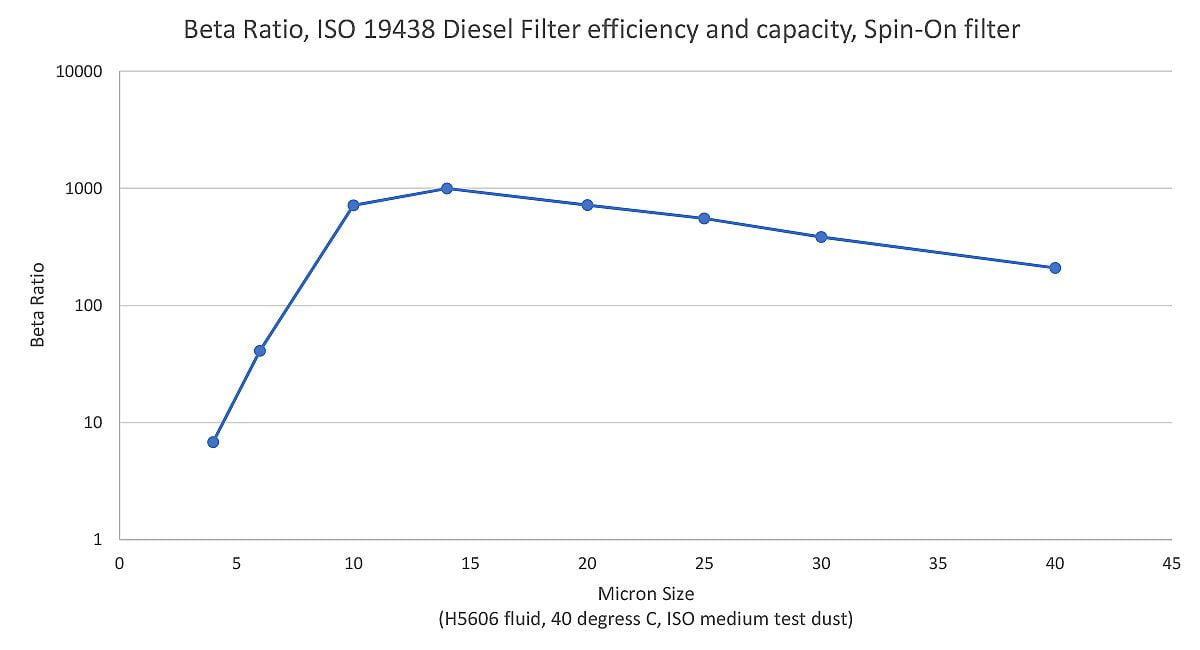What is a Micron?

The word micron is another term for micrometer (1 millionth of a meter). It is used like the inch, foot, centimeter, and millimeter to measure length, width or diameter of objects. Its scientific notation is μ or μm. One inch is 25,400 microns. Some comparative sizes from largest to smallest are:

The diameter of average human hair = 70 microns
The lower limit of visibility (naked eye) = 40 microns
White blood cells = 25 microns
Talcum powder = 10 microns
Red blood cells = 8 microns
Bacteria = 2 microns
Tobacco smoke = 0.5 microns

What does a filter micron rating really mean?

A hydraulic filter element filter rated “10 micron” has some capability to capture particles as small as 10 microns. However, when you see a hydraulic filter marked “10 micron”, you do not know exactly what this means unless you also have a description of the test methods and standards used to determine the filter rating. The results from the different test methods may not be comparable as their methodology varies greatly.

The two most popular reported media ratings are a nominal micron rating (50+% efficiency) and an absolute micron rating (98.7+% efficiency). A nominal rating usually means the filter’s media can capture a given percentage of particles of stated size. For example, a hydraulic filter might be said to have a nominal rating of 50% for particles 10 micrometers in size or larger. An absolute micron rating is determined by single-pass or multi-pass testing. The absolute rating is obtained by passing a test fluid containing particles of a known size through a small, flat sheet of filter media. Any particles that pass through the media measured and compared to the number of particles in the unfiltered fluid.  An absolute rating is also expressed as a percentage of the size of particles captured.

What is a Beta Ratio?

A test procedure called multi-pass testing, or Beta ratio testing (β), is a universally accepted test method that yields readily comparable test results. Multi-pass testing has been recognized by SAE (Society of Automotive Engineers SAE J1858), ISO (International Organization of Standardization ISO 4548-12, lube oil, and ISO16889, hydraulic or fuel), ANSI (American National Standards Institute) and NFPA (National Fluid Power Association).

From upstream and downstream particle counts, a Beta ratio is formulated by dividing the number of particles of a particular size in the upstream flow by the number of particles of the same size in the downstream flow.  Beta ratios are written as “Bx = Y”, where X is the micron size and Y is the Beta Ratio, for example, B10 = 75.Can you convert a Beta Ration into easy-to-understand information?

To translate the Beta ratio into easy-to-understand information, subtract 1 from the original ratio and divide that answer by the original ratio. This answer represents the efficiency of the media at the specified particle size.

For example, B10=75, take the Beta ratio of 75, subtract 1 from it and divide that answer by the original ratio of 75, or 75 – 1 = 74 ÷ 75 = 98.66% efficient at removing 10 micron-sized particles.

This formula is used to translate any Beta ratio into a percent efficiency at removing the size of particle tested.  Both equations state the same information in two different ways and are both accepted by the industry.

What is a filter’s Dirt Holding Capacity

The ability of an element to retain dirt or contaminant is referred to as its “Dirt Holding Capacity”. It determines the life of the element and the length of service it will perform before it becomes clogged to the point it must be replaced.

What is a filter Dirt Capacity Test?

The dirt capacity test determines the weight of a specified artificial contaminant which must be added to the influent to produce a given differential pressure across a filter at a specified condition. It is used as an indication of relative service life. The test result is usually stated in grams.

What is Pressure Drop (Differential Pressure, or Delta P)?

Pressure Drop is the difference in system pressure between the upstream and downstream sides of the filter.
Four Major Factors Contribute to Pressure Drop:

1. Filter Media
2. Dirt Contamination
3. Flows (higher flows create higher pressure drop)
4. Fluid Viscosity (higher fluid viscosity’s mean higher pressure drop)